Home » Siemens PLC Mathematics Instructions
PLC Tutorials

# Siemens PLC Mathematics Instructions

This is Program for Siemens PLC Mathematics Instructions.

## Siemens PLC Math Instructions

Implement ADD, SUB, MUL, DIV, MOD and NEG instructions in S7-1200 PLC using ladder diagram language.

### Problem Diagram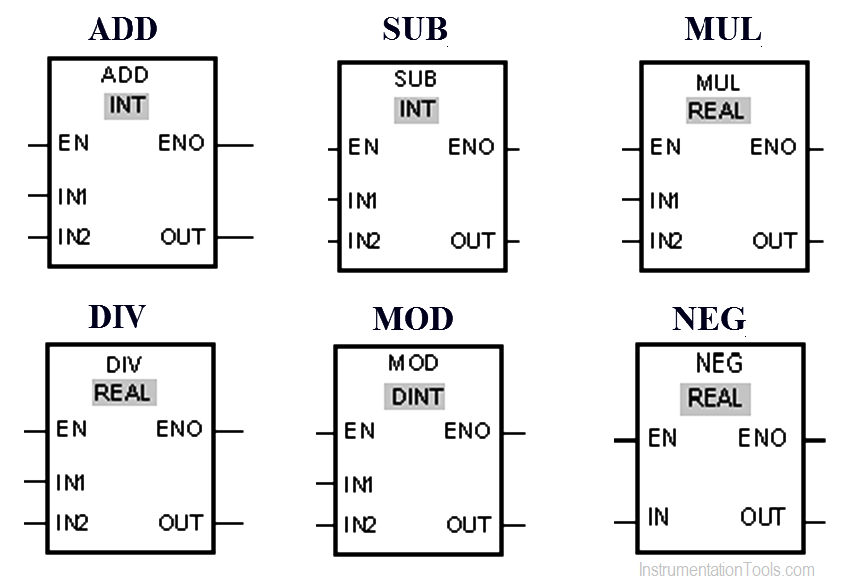### Solution

We can use the Add instruction to add the value at input IN1 and the value at input IN2 and query the sum at output OUT (OUT := IN1+IN2).

Subtract instruction is used to subtract the value at input IN2 from the value at input IN1 and query the difference at output OUT (OUT := IN1-IN2).

Multiply instruction is used to multiply the value at input IN1 with the value at input IN2 and query the product at output OUT (OUT := IN1*IN2).

Divide instruction is used to divide the value at input IN1 by the value at input IN2 and query the quotient at output OUT (OUT := IN1/IN2).

We can use the Return remainder of division instruction to divide the value at input IN1 by the value at input IN2 and query the remainder of division at output OUT.

We can use the “Create twos complement” instruction to change the sign of the value at the IN input and query the result at the OUT output. If there is a positive value at input IN, for example, the negative equivalent of this value is sent to output OUT.

### List of Inputs/Outputs

#### M memory

• MW0 :- “ADD_VALUE 1”
• MW2 :- “ADD_VALUE 2”
• MW4 :- “ADD_RESULT”
• MW6 :- “SUB_VALUE 1”
• MW8 :- “SUB_VALUE 2”
• MW10 :- “SUB_RESULT”
• MW12 :- “MUL_VALUE 1”
• MW14 :- “MUL_VALUE 2”
• MW16 :- “MUL_RESULT”
• MW18 :- “DIV_VALUE 1”
• MW20 :- “DIV_VALUE 2”
• MW22 :- “DIV_RESULT”
• MW24 :- “NEG_VALUE”
• MW226 :- “NEG_RESULT”
• MD100 :- “MOD_VALUE 1”
• MD104 :- “MOD_VALUE 2”
• MD108 :- “MOD_RESULT”

### PLC Ladder diagram for mathematical instructions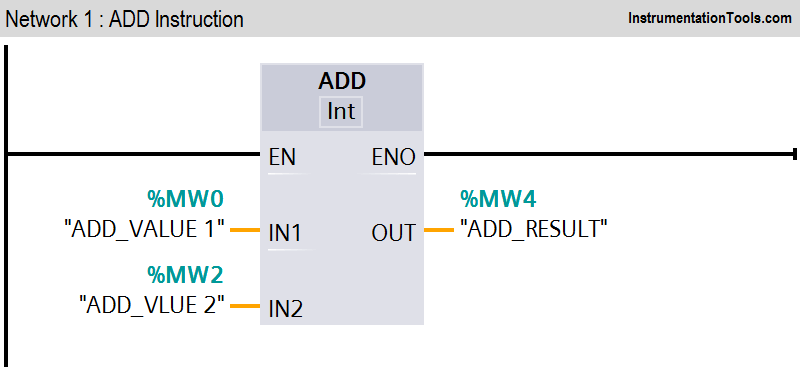###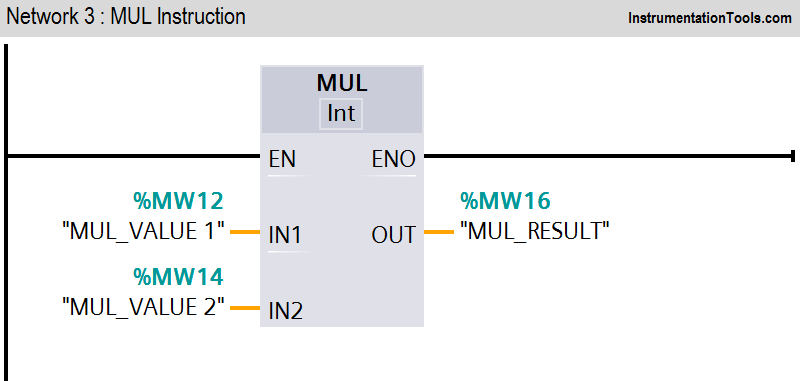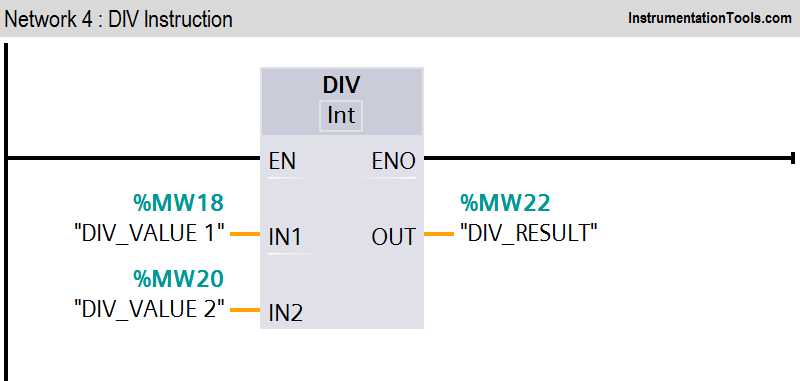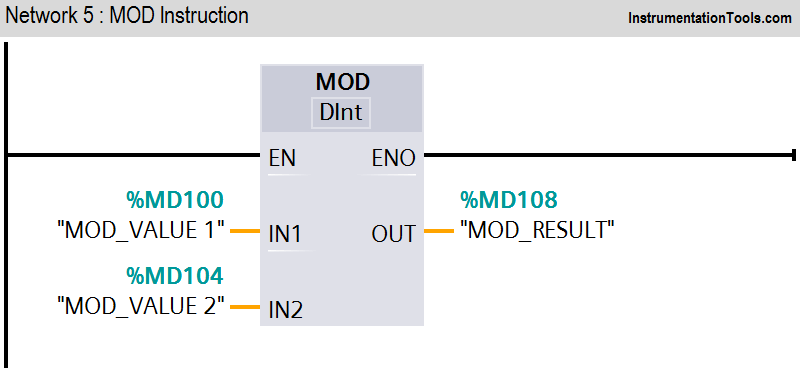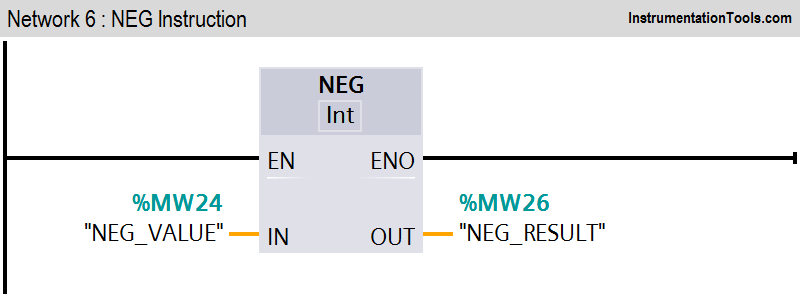Program Description

In this program we have used Siemens S7-1200 PLC and TIA Portal Software for programming.

#### Network 1 :-

The value of MW0 is added to the value of MW2.The result of the addition is stored in the MW4.

#### Network2 :-

The value of MW6 is subtracted from the value of MW8.The result of the subtraction is stored in MW10.

#### Network 3 :-

The value of operand MW12 is multiplied by the value of MW14. The result of the multiplication is stored in MW16.

#### Network 4 :-

The value of operand MW18 is divided by the value of MW20. The division result is stored in MW22.

#### Network 5 :-

The value of MD100 is divided by the value of MD104. The remainder of division is stored in operand MD108.

#### Network 6 :-

The sign of the value at input MW24 is changed and the result is provided at output MW26.

#### Light ON OFF Control using PLC

This website uses cookies to improve your experience. We'll assume you're ok with this, but you can opt-out if you wish. Accept Read More

WordPress Image Lightbox

Send this to a friend